Do you like the article?
Share it with others -

Use new possibilities of MetaTrader 5

# How to Write Fast Non-Redrawing ZigZags

12 September 2008, 10:516
5 743

### Introduction

Among all possible algorithms of zigzag charting, we can distinguish a class that the author names "Zigzags with Switching upon Breaking Through the Slowing Level". This class, in full or in part, includes most existing ZigZags. The name of the class itself, in fact, represents an algorithmic template. To make an indicator out of it, it is sufficient just to add the function that would detect the slowing level. The diversity of algorithms of such function is only limited by the imagination of the author of the future ZigZag.

### General Approach

First of all, let's try to formulate the general approach to writing an indicator. Thus:

- Function start() of any indicator (as well as any EA) represents a callback function, i.e., a function to be called to process a specific event. Namely, to process a tick.

- The object of writing an indicator is, as a rule, the calculation of one or several market characteristics. Along with the ancillary quantities necessary for calculations, they form a set of key variables of the given indicator. Let's defined the state of the indicator as a set of the values of those key variables at a specific time. Basing on this definition, we can state the following:

• Calculating the new values of variables at a new tick, function start() calculates the new state of the indicator.
• Thus, in fact, function start() is an operator that transfers the indicator from one state into another.

- In these terms, the process of writing an indicator reduces itself to determining a set of quantities describing its state (state variables) and to writing an operator that would transfer the indicator into a new state at the arrival of a new tick. Initialization of state variables becomes an essential part of the indicator algorithm. We will show how all this can be done at the example of ZigZags of a certain type.

### What ZigZags Are in Question

As it was said above, in this article, we are interested in ZigZags switching at breaking through the slowing level. What is a "slowing level"? Assume we want to write a ZigZag for which the peak is fixed when the price moves from that peak by H points. Fixing a peak means switching the direction of a ZigZag segment to an opposite one. Let us have just fixed the minimum and now be in an up-segment. Let's introduce a variable for the price time maximum of an incomplete up-segment, TempMax. We will fix this maximum (and switch the direction), if the price breaks through the level of:

SwitchLevel = TempMax - H *Point .

If the time maximum is updated before switching, we will have to calculate the new value of SwitchLevel. Thus, SwitchLevel will follow the time maximum, being H points behind it.

The situation will be absolutely symmetric for a down-segment: SwitchLevel will now follow the time minimum (TempMin ), being the same H points behind it. But now, we will have:

SwitchLevel = TempMin + H *Point .

In fact, we have just described the algorithm of calculating the slowing level for the ZigZag we are going to create. Obviously, it is not the only possible algorithm. For example, if we consider the lower/upper boundary of a channel to be the slowing level, we will get exactly as many ZigZags as there are methods of channel calculation. Moreover, at a closer consideration, the absolute majority of ZigZags known by the author turn out to be fully or partly included into the class under consideration. But not all of them. For example, the ZigZag calculated on Williams' fractals cannot be included into this class.

### ZigZag Model

Let's now determine the variables of the ZigZag state.

First of all, it will be the direction of the current segment. We will name the corresponding variable UpZ and assign it the values of true for up-segments and false for down-segments.

Obviously, we should add to the list TempMax and TempMin introduced above. We will also add their time coordinates. Here, however, we get some degree of freedom in defining the units of measurement. As a time coordinate, we will use the bar number starting from the beginning of the chart, i.e., we will use the numbering system being reverse to that accepted in MT4. This will both simplify the code and enhance its execution rate. Thus, the list will be replenished with variables TempMaxBar and TempMinBar.

We plan both draw the ZigZag on a chart and use it somehow. So we will add to the list the coordinates of the last fixed ZigZag peaks: CurMax, CurMaxBar, CurMin, CurMinBar.

And that's that for the list. An individual author of a specific ZigZag can freely replenish the list with the things he/she is going to do with this ZigZag. For example, it may turn out reasonable to add the coordinates of the preceding peaks: PreMax, PreMaxBar, PreMin, PreMinBar. Or you may need to add the coordinates of a predefined number of preceding peaks, using arrays, in such case.

### Transition Operator

In the proposed approach, writing a transition operator for a ZigZag becomes a rather simple task. We have just to translate the definition of the ZigZag class we are interested in into MQL4. This is how it will appear:

```// First, process the case of an up-segment
if (UpZ) {
// Check whether the current maximum has changed
if (High[pos]>TempMax) {
// If yes, then correct the corresponding variables
TempMax = High[pos];
TempMaxBar = Bars-pos;  // Here switching to direct numbering
} else {
// If not, then check whether the slowing level has been broken through
if (Low[pos]<SwitchLevel()) {
// If yes, then fix the maximum
CurMax = TempMax;
CurMaxBar = TempMaxBar;
// And draw a peak
ZZ[Bars-CurMaxBar]=CurMax;  // Here switching to reverse numbering
// Correct the corresponding variables
UpZ = false;
TempMin = Low[pos];
TempMinBar = Bars-pos;  // Here switching to direct numbering
}
}
}  else {
// Now processing the case of down-segment
// Check whether the current minimum has changed
if (Low[pos]<TempMin) {
// If yes, then correct the corresponding variables
TempMin = Low[pos];
TempMinBar = Bars-pos;  // Here switching to direct numbering
} else {
// If not, then check whether the slowing level has been broken through
if (High[pos]>SwitchLevel()) {
// If yes, then fix the minimum
CurMin = TempMin;
CurMinBar = TempMinBar;
// And draw a peak
ZZ[Bars-CurMinBar]=CurMin;  // Here switching to reverse numbering
// Correct the corresponding variables
UpZ = true;
TempMax = High[pos];
TempMaxBar = Bars-pos;  // Here switching to direct numbering
}
}
}```

The transition operator is ready. Now we can refer to state variables of the indicator at any time.

However, such operator has a special feature that can be perceived as an error drawing the ZigZag. Let's consider the fragment below in more details:

```      if (High[pos]>TempMax) {
// If yes, then correct the corresponding variables
TempMax = High[pos];
TempMaxBar = Bars-pos;  // Here switching to direct numbering
} else {
// If not, then check whether the slowing level has been broken through
if (Low[pos]<SwitchLevel()) {
// If yes, then fix the maximum
CurMax = TempMax;
CurMaxBar = TempMaxBar;```

Using the pair of if - else means that the Low of the bar containing TempMax will not be considered. The situations where this price turns out to be below the next fixed minimum can be perceived as error drawing the ZigZag. Is it an error, indeed?

Considering that the working on history and in real time must be identical, the author holds the opinion that this is not an error. Indeed, inside a timeframe, we will never know what happened on history earlier, the bar maximum or minimum. Using here the construction of if - else means making a conscious decision: We prefer momentum. It means that we sacrifice the minimum for an up-segment and the maximum for a down-segment. It stands to reason that the smaller the timeframe is the less frequently this dilemma will occur.

```// Correct the corresponding variables
UpZ = false;
TempMin = Low[pos];
TempMinBar = Bars-pos;  // Here switching to direct numbering
}
}```

Here, in fact, the point of starting the time minimum check is set for the moment of switching between segments. It is justifiable for the given example, but, in general case, you must not do so. It would be more reasonable to set the temporary minimum for the minimal interval from the fixed maximum to the current position (i.e., to the moment of switching). The code can be as follows:

```   // Correct the corresponding variables
UpZ = false;
TempMinBar = CurMaxBar+1;
TempExtPos = Bars - TempMinBar;  // Here switching to reverse numbering
TempMin = Low[TempExtPos];
for (i=TempExtPos-1;i>=pos;i--) {
if (Low[i]<TempMin) {
TempMin = Low[i];
TempMinBar = Bars-i;  // Here switching to direct numbering
}
}```

Here, the Low of the bar where the minimum has been fixed is excluded from consideration again.

These two notes also concern processing down-segments.

### Indicator

It only remains to complete the indicator to make it working. There is no need to comment on init() and deinit(), everything is pretty clear and standard there. However, we will make an important decision on function start(). We will work with complete bars only. The main reason for this is that it allows us to achieve a simple and compact code structure.

There is another important consideration about that. A serious work on a trading system implies collecting statistics on history. This statistics will be valid (correct) only if the characteristics obtained in real time completely correspond with those obtained on history. We don't have a history of real ticks, so we can only achieve that full compliance working in real time with complete bars only. The maximum we can do to reduce the delay is to go to smaller timeframes, down to M1.

Another essential feature is not to use function IndicatorCounted(). The main reason for doing so is that the code used needs another important action - initialization of state variables of the indicator. This cannot be done in function init() since using direct numbering requires to recalculate the indicator at history pumping and, therefore, to re-initialize state variables. Function init() is not launched at history pumping.

Thus, we have to add one more "standard" function, Reset(). Eventually, the wish to use IndicatorCounted() not so much helps as hinders to organize recalculation check necessary for an indicator of this type. This check is realized as follows:

```int start() {
//  Work with completed bars only
if (Bars == PreBars) return(0);
//  Check whether there are enough bars on the chart
if (Bars < MinBars) {
Alert(": Not enough bars on the chart");
return(0);
}
//  If the history was not pumped, make calculations for the bar just completed
if (Bars-PreBars == 1 && BarTime==Time) StartPos = 1;
//  Otherwise, count the number of bars specified in function Reset()
else StartPos = Reset();
// Modify check variables
PreBars = Bars;
BarTime=Time;
// Cycle on history
for (pos=StartPos;pos>0;pos--) {```

Function Reset() appears as follows:

```int Reset() {
if (MinBars == 0) MinBars = Bars-1;
StartPos = MinBars;
PreBars = 0;
BarTime = 0;
dH = H*Point;
UpZ = true;
TempMaxBar = Bars-StartPos;
TempMinBar = Bars-StartPos;
TempMax = High[StartPos];
TempMin = Low[StartPos];
StartPos++;
return(StartPos);
}```

Here we could pay a special attention to the additional variable, dH, to which we once for all assign the ZigZag switch threshold value (H ) transformed into price scale. A question may arise: Why is UpZ = true, not false ? The answer is simple: After a small number of segments, the indicator will result in the same graph, independently on the initial value of UpZ.

Well, finally, the calculations of the slowing level:

```double SwitchLevel() {
double SwLvl;
if (UpZ) SwLvl = TempMax - dH;
else SwLvl = TempMin + dH;
return(SwLvl);
}```

Everything must be clear here.

### Conclusion

A template for writing ZigZags, ZZTemplate, is attached to this article. All you have to do is to add the necessary code to function SwitchLevel(). To turn the template into the ZigZag used here as an example, you have just to find the following lines and comment on them:

`//extern int H = 33;`
`//double dH;`
`//  dH = H*Point;`
```//  if (UpZ) SwLvl = TempMax - dH;
//  else SwLvl = TempMin + dH;```

The final note concerns the ZigZag speed. The template implies generality. Besides, we want to have as transparent structure as possible. I think most specific realizations can be additionally optimized to enhance its operation.

The general recommendation is as follows: Where possible, place operations into if operators. As an example (but not an ideal model) of optimization, please find attached indicator HZZ, an alternative realization of the ZigZag used in this article. The simplicity of the problem allows us to abandon both function SwitchLevel() and some state variables. As a small bonus, I added to HZZ writing ZigZag peaks to file and "on-the-fly" checking some statistical characteristics of ZigZag.

Translated from Russian by MetaQuotes Software Corp.
Original article: https://www.mql5.com/ru/articles/1545

Attached files |
HZZ.mq4 (4.22 KB)
ZZTemplate.mq4 (5.67 KB)
Last comments | Go to discussion (6)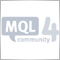| 24 Jun 2009 at 10:02
Thanks a lot for this one, it realy helped me!| 8 Oct 2009 at 03:56
heelo candid,Thanks a million for this great article.please i would like to know how i can add codes to get the coordinates of a predefined number of preceding peaks, using arrays like you suggested.i need to get the values of the last three peaks.Thanks a million| 1 Nov 2012 at 11:08
best timeframe for zig zag to use..reply??| 22 Nov 2012 at 18:21

Hi guys,

I can translate from 'ru' to 'en' the comments, if needed, give me  about 2 or 3 hours. Interested ?forextime8 | 31 Aug 2014 at 08:19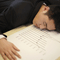Idleness is the Stimulus to Progress, or How to Work with Graphics Interacively

An indicator for interactive working with trend lines, Fibo levels, icons manually imposed on a chart. It allows you to draw the colored zones of Fibo levels, shows the moments of the price crossing the trend line, manages the "Price label" object.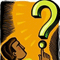Changing the External Parameters of MQL4 Programs without Restarting

The article describes a method of changing the external parameters of MQL4 programs on-the-fly, without restarting.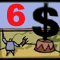Expert Advisors Based on Popular Trading Strategies and Alchemy of Trading Robot Optimization (Cont.)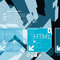HTML Walkthrough Using MQL4